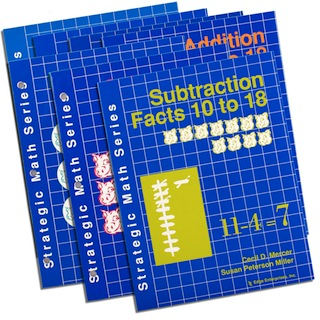So you’ve tried everything you could think of, from flash cards to nightly homework, but those basic math facts still aren’t sinking in. Solve this problem now with the Strategic Math Series, the student-approved series that’s been adopted by the Sylvan Learning Center—because it works! The series consists of seven books: Addition Facts 0 to 9, Subtraction Facts 0 to 9, Addition Facts 10 to 18, Subtraction Facts 10 to 18, Place Value: Discovering Tens and Ones, Multiplication Facts 0 to 81, and Division Facts 0 to 81.

Each book in this popular series is based on the “concrete-representational- abstract” method of instruction. Basically, this means that students first learn to understand a math fact by using concrete objects, then move to pictures like dots or tallies that represent a certain number of objects, and finally, they work with numbers alone.

Within each level of instruction, students also learn to solve the ever-dreaded “word problems,” using a simple strategy. Each book comes with 21 learning sheets for students to practice specific facts and word problems, a pair of cheerful “pig dice” for playing math games in later lessons, and special pages that enable them to practice mixed facts (i.e., addition, subtraction, multiplication, and division) and increase their speed at coming up with the correct answers. This series can be used either as a basic math curriculum for grades 1-6, or as a remedial program for older students who still need to learn basic facts.

These books are now being distributed through the University of Kansas Center for Research on Learning at 785-864-4780.

• Sort by Default
• Display 15 Products per page